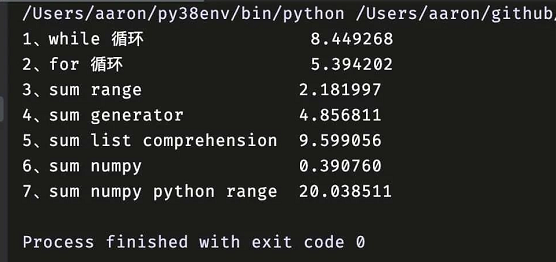2022
08-04

# Python中最快的循环方式是哪一种？#### 1、while 循环

```def while_loop(n=100_000_000):     i = 0     s = 0     while i < n:
s += i
i += 1     return s```

#### 2、for 循环

```def for_loop(n=100_000_000):     s = 0     for i in range(n):
s += i
return s```

#### 3、sum range

`def sum_range(n=100_000_000):     return sum(range(n))`

#### 4、sum generator（生成器）

`def sum_generator(n=100_000_000):     return sum(i for i in range(n))`

#### 5、sum list comprehension（列表推导式）

`def sum_list_comp(n=100_000_000):     return sum([i for i in range(n)])`

#### 6、sum numpy

`import numpy def sum_numpy(n=100_000_000):     return numpy.sum(numpy.arange(n, dtype=numpy.int64))`

#### 7、sum numpy python range

`import numpy def sum_numpy_python_range(n=100_000_000):     return numpy.sum(range(n))`

```import timeit def main():     l_align = 25     print(f'{"1、while 循环":<{l_align}} {timeit.timeit(while_loop, number=1):.6f}')
print(f"{'2、for 循环':<{l_align}}  {timeit.timeit(for_loop, number=1):.6f}")
print(f'{"3、sum range":<{l_align}} {timeit.timeit(sum_range, number=1):.6f}')
print(f'{"4、sum generator":<{l_align}} {timeit.timeit(sum_generator, number=1):.6f}')
print(f'{"5、sum list comprehension":<{l_align}} {timeit.timeit(sum_list_comp, number=1):.6f}')

print(f'{"6、sum numpy":<{l_align}} {timeit.timeit(sum_numpy, number=1):.6f}')

print(f'{"7、sum numpy python range":<{l_align}} {timeit.timeit(sum_numpy_python_range, number=1):.6f}') if __name__ == '__main__':
main()```#### for 比 while 块

for 和 while 本质上在做相同的事情，但是 while 是纯 Python 代码，而 for 是调用了 C 扩展来对变量进行递增和边界检查，我们知道 CPython 解释器就是 C 语言编写的，Python 代码要比 C 代码慢，而 for 循环代表 C，while 循环代表 Python，因此 for 比 while 快。

#### numpy 内置的 sum 要比 Python 的 sum 快

numpy 主要是用 C 编写的，相同的功能，肯定是 numpy 的快，类似的，numpy 的 arange 肯定比 Python 的 range 快。

#### 交叉使用会更慢

numpy 的 sum 与 Python 的 range 结合使用，结果耗时最长，见方法 7。最好是都使用 numpy 包来完成任务，像方法 6。

#### 最后